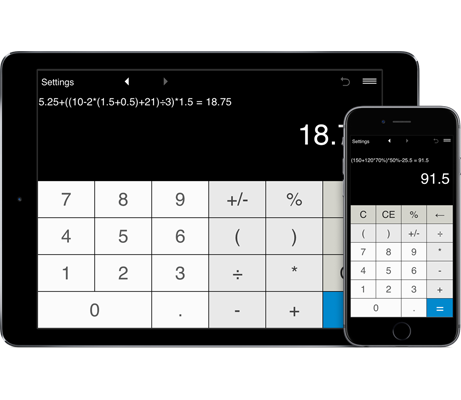# Calculator with parentheses

Basic calculations: addition, subtraction, multiplication and division.
Calculations with parentheses, including nested parentheses.
Percentage calculations.
History tape to view recent calculations.

## How you can use the calculator with parentheses

This simple calculator is primarily intended to help people in basic calculations. You can access the calculator from any computer or mobile device.

The basic calculator offers four functions, which include addition, subtraction, multiplication and division. Unlike many other calculators, this calculator supports expressions with parentheses, including nested parentheses.

The calculator can be used to perform calculations with percentages. You can also use the calculator to convert fractions to decimals. For example, if you have the fraction 1/4, press 1 ÷ 4 = and the calculator will show the result 0.25.

The calculator options can be accessed by clicking on the buttons or using the keyboard.

The calculator stores history of recent calculations.

Back and forward buttons lets you check and use recent calculations.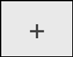Adds.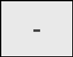Subtracts.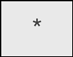Multiplies.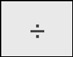Divides.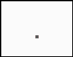Inserts a decimal point.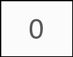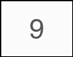Puts the number in the calculator's display.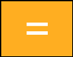Performs any operation on the previous two numbers. To repeat the last operation, you should click = again.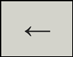Deletes the last digit of the displayed number.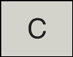Clears the current calculation.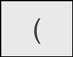Starts a new level of parentheses. The maximum number of levels is 100.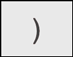Closes the current level of parentheses.

### Using the calculator to find percentages

This calculator is very useful when making percentage calculations. To calculate percentages with this calculator, you should use the "%" button.

#### Percentage calculations:

1. Converting fractions to percentages with a calculator.
Use a calculator to convert any fraction to a percentage.
To change 1/8 into a percentage, just type the buttons 1 ÷ 8 % =
The calculator will now show the value of 12.5 (12.5%).
For example, if you have the fraction 1/4, press 1 ÷ 4 % =
The result is 25 (25%).
Useful fractions to percentages conversions:
1/2 = 50%
1/3 = 33 1/3%
1/4 = 25%
1/5 = 20%
1/8 = 12.5%
3/4 = 75%
Example of percentage calculation: 8 out of 120 is what %?

2. Converting percentages to decimals with a calculator.
With this basic calculator you convert any number from its percentage form to its decimal form. Just enter the number and press the % button, and the calculator will show the decimal equivalent.
For example, if you have the decimal 2.5, press 2.5 %
and the calculator will show the result 0.025.
To represent 15 % on your calculator, just press 15 and then %. The calculator will turn 15 % into 0.15, which is the decimal equivalent.

4. How to find the percent of a number.
To find the percent of a number, you should performing multiplication. Let’s say you want to find 25% of 94. If you want to find the percent of a number, the word "of" means you need to multiply. Therefore, 25% of 94 means 25% x 94.
To input the problem press the following buttons:
94 * 25 % =
or 25 % * 94 =
As you enter the number and then %, as soon as your hit the % button, your number 25 converts to 0.25 and the calculator will calculate 0.25 x 94.
Example of percentage calculation: what is 12% of 80?

5. How to add and subtract percentages with the calculator
Examples of percentage calculation:
The initial price \$20.00 was increased by 35%. What was the new price?
To solve this problem, type 20.00 + 35% =
and the calculator will show the result 27.

The initial price \$49.00 was decreased by 70%. What was the new price?
49.00 - 70% =
and the calculator will show the result 14.7.